# Exterior product

A fundamental operation in the exterior algebra of tensors defined on an-dimensional vector spaceover a field.

Let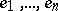be a basis of, and letandbe- and-forms: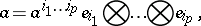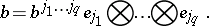The exterior product of the formsandis the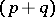-formobtained by alternation of the tensor product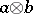. The formis denoted by; its coordinates are skew-symmetric: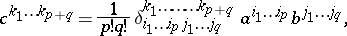where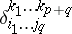are the components of the generalized Kronecker symbol. The exterior product of covariant tensors is defined in a similar manner.

The basic properties of the exterior product are listed below:

1)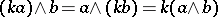,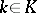(homogeneity);

2)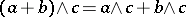(distributivity);

3)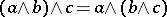(associativity).

4)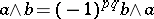; if the characteristic ofis distinct from two, the equation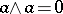is valid for any formof odd valency.

The exterior product ofvectors is said to be a decomposable-vector. Any poly-vector of dimensionis a linear combination of decomposable-vectors. The components of this combination are the (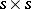)-minors of the (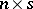)-matrix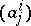,,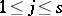, of the coefficients of the vectors. If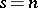their exterior product has the formOver fields of characteristic distinct from two, the equationis necessary and sufficient for vectors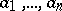to be linearly dependent. A non-zero decomposable-vectordefines inan-dimensional oriented subspace, parallel to the vectors, and the parallelotope informed by the vectors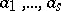issuing from one point, denoted by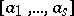. The conditions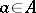and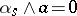are equivalent.

For references see Exterior algebra.

Instead of exterior product the phrase "outer product" is sometimes used. The conditionforof degreeandof degreeis sometimes called graded commutativity.